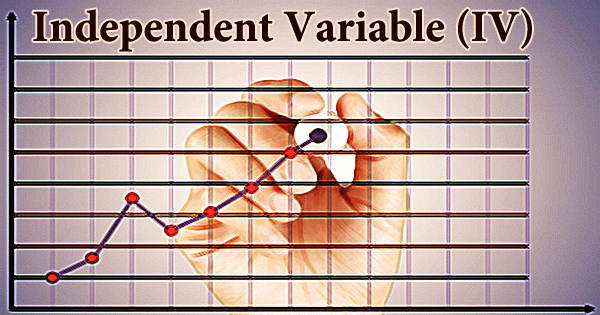Finance

# Independent Variable (IV)An input, assumption, or driver that is modified in order to analyze its influence on a dependent variable (the outcome) is known as an independent variable (IV). It is a feature of a psychological experiment that is modified or altered by researchers rather than by other factors in the experiment. An analyst often does sensitivity analysis in Excel in financial modeling and analysis, which includes altering assumptions in the model to see how they affect the result.

In an experiment, the dependent variable is the variable that is being tested and measured, and it is ‘depending’ on the independent variable. Independent variables, on the other hand, are not viewed as being reliant on any other variable in the experiment at hand. For example, studying would be the independent variable in a study of the impact of studying on test results. The goal of the study is to see if changing the independent variable causes substantial changes in the dependent variable.

In the assumptions area of an Excel model, an analyst must carefully consider how to put up an independent variable. They are the inputs that will be used to drive the model and its outputs. A root-cause analysis is one of the most effective techniques to choose assumptions. Time, space, density, mass, fluid flow rate, and historical values of any observable value of interest (e.g. human population number) are some typical independent variables used to forecast future values (the dependent variable).

The independent variable is one that the researchers either control (for example, the amount of something) or that already exists but is unaffected by other factors (such as the age of the participants). The next step is to build formulae and functions that determine the outputs after the variables have been identified and set up in Excel. Total revenue, for example, is the number of stores multiplied by the number of goods sold per store multiplied by the average price per product.

When the independent variable changes, the dependent variable changes as well. The influence on the dependent variable is calculated and documented. Changes in any of the underlying assumptions and drivers will affect the dependent variable, revenue, once the function has been constructed. The independent variable is plotted on the x-axis, while the dependent variable is recorded on the y-axis when graphing data for an experiment.

The independent variables in a given experiment are all determined by the hypothesis and the question the experimenters are attempting to answer. Some tests should be run to check that the linking is correct and that the dependant variable changes as anticipated when the assumptions change. An independent variable in an experiment is any variable that the experimenter manipulates.

Models and experiments are used to investigate the impact of independent factors on dependent variables. After the model has been constructed, you may begin adjusting assumptions and drivers to observe how they affect the dependent variable, as well as test hypotheses, run scenarios, and assess sensitivity. Independent variables are also included for additional purposes, such as to account for their possible confounding impact, even though their influence is not directly of interest.

Keep in mind that the independent variable is the one that the researcher manipulates in order to determine its impact on the dependent variable. An independent variable is a symbol that represents an arbitrary input, whereas a dependent variable is a symbol that represents an arbitrary output. The most frequent input symbol is x, and the most common output symbol is y; the function is often represented as y = f(x). One set of workers performs a job in a yellow room, while another does the identical activity in a blue room, as part of an experiment. The color of the workplace is the independent variable in this case.

Even though there is only control and an experimental group, the independent variable varies in every experiment. A company wants to know if providing workers more choice over how they conduct their jobs increases job satisfaction. One set of workers is given a lot of say in how they do their work in an experiment, whereas the other group isn’t. In this case, the independent variable is the degree of control the workers have over their job.

Changing the independent factors can sometimes lead to changes in the dependent variables. In other situations, researchers may discover that changes in the independent variables have no influence on the variables under study. Even though there is only control and an experimental group, the independent variable varies in every experiment. In reaction to the independent variable, the dependent variable may or may not change.

Information Sources: